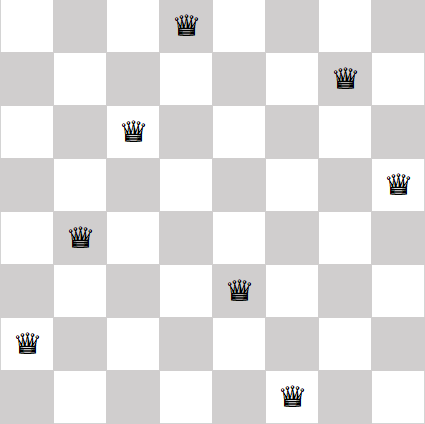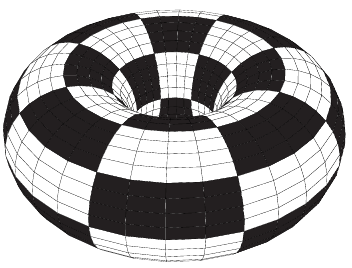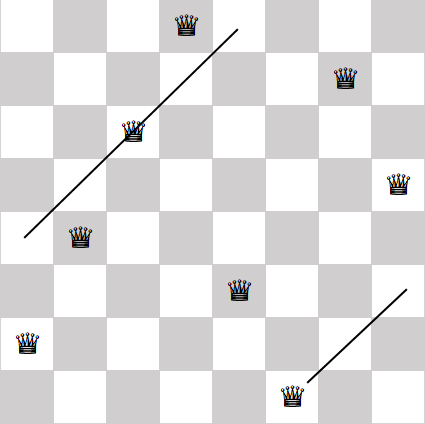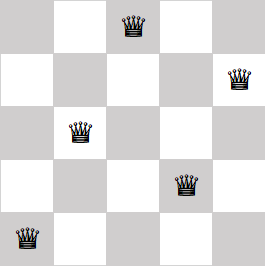# Queens on a donut

The eight queens problem is to place eight queens on a chessboard so that no queen attacks another. Because queens are allowed to move any number of spaces horizontally, vertically, or diagonally, this means no queen can be on the same row, column, or diagonal as any other queen.

For example, the following image gives one solution to the eight queens problem. (See the previous post for how I made the chessboard images in this post.)The eight queens problem has been generalizes many ways, first by considering square chessboards of different sizes. The n-queens problem works on an n by n board. The n-queens problem can be solved for all n greater than 3.

The problem I want to consider here is the toroidal n queens problem. We first curl up our chessboard into a cylinder, then curl join the ends of the cylinder together to make a torus (donut).(I found the image above on TeX StackExchange.)

When we do turn our chessboard into a torus, the rows and columns don’t change. If no two queens were on the same row (column) on the flat chessboard, there still won’t be any two queens on the same row (column) in the toroidal chessboard.

But the diagonals do change, as the following image illustrates.If we curl our chessboard vertically by joining the left and right edges—no need to imagine the torus for this example, a cylinder will do—then some of the diagonals merge. The queen on the bottom row would be on the same diagonal as the queen in the third row from the top.

George Pólya proved that the toroidal n queens problem has a solution if and only if n is not a multiple of 2 or 3. Not only does the particular solution to the eight queens problem above not extent to a torus, no solution to the eight queens problem extends to a torus because 8 is divisible by 2.

The smallest non-trivial chessboard of size n with n not divisible by 2 or 3 is n = 5. The following solution to the five queens problem remains a solution if you identify the edges, making the board into a torus.## 2 thoughts on “Queens on a donut”

1. Note that for n odd, some diagonal moves change the color of the squares as the piece is moved.

2. In retrospect very surprised that the computer scientist and professor Boris Stilman (perhaps still at the University of Colorado?) never used these Queens examples in his explication of his “linguistic geometry” which started with chess and then used that as a metaphor to build up tiers of grammars of reachability for board games (generally wargames).